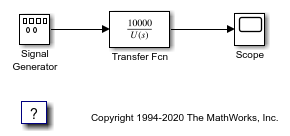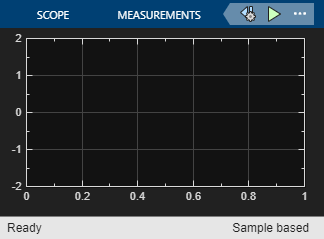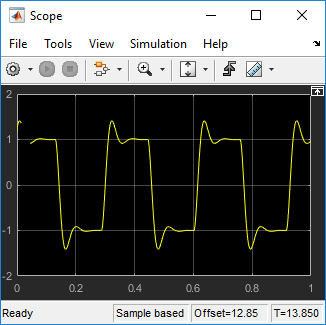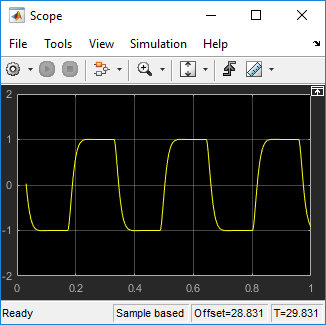# Sweep MATLAB Variables with MATLAB Scripting

This example shows you can embed MATLAB® variables in the base workspace with MATLAB commands and use MATLAB language to change their values during execution of Simulink® Desktop Real-Time® models.

In Connected IO mode or accelerator mode, Simulink® transfers the new values to the model that is being simulated. In Run in Kernel mode, Simulink transfers the new values to the real-time application that is running in the kernel mode process.

### Example Goal

For this example, your goal is to minimize ringing in the transfer function. For improved performance, you have inlined block parameters. When you inline block parameters, the parameters appear as nontunable constants in the generated code. To make an individual parameter tunable, use a MATLAB variable with a storage class other than Auto to store the parameter in memory.

You can permanently store parameter objects and other external data in a data dictionary.

### Open the Model

This procedure uses the square-wave transfer function model sldrtex_inlined.

Install the Simulink Desktop Real-Time kernel and change to a working folder.

1. Open `sldrtex_inlined` and the `Scope` block.

```model = 'sldrtex_inlined'; open_system(model); scname = [model '/Scope']; open_system(scname) ```2. In the base workspace, create a parameter object configured to store the parameter as a global variable.

```Dmp = Simulink.Parameter([1 70 10000]); Dmp.StorageClass='ExportedGlobal';```

3. Replace the `Transfer Fcn` block parameter `Denominator` with the parameter object.

```xfername = [model '/Transfer Fcn']; set_param(xfername,'Denominator','Dmp');```

4. Start execution with the original Dmp variable value.

```set_param(model,'StopTime','Inf'); set_param(model,'SimulationMode','external') set_param(model,'SimulationCommand','connect') set_param(model,'SimulationCommand','start')```

*5.*Sweep the Dmp variable from 30 to 180 by 30.

```for Val = 30 : 30 : 180 Dmp.Value = [1 Val 10000]; set_param(model,'SimulationCommand','update')```
``` pause(2.0) end```

### Observe Signals on the Scope

The Scope block shows changes at 30-unit intervals. The figures show key changes.

### Figure Val == 30### Figure Val == 90### Figure Val == 180### Stop Execution

`set_param(model,'SimulationCommand','stop');`

### Close Model

To close the model, in the MATLAB Command Window, type:

```bdclose("all"); ```